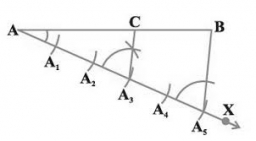# Divided 71124

We divided the line AB into two parts in a ratio of 3: 5. The longer part was 6 cm longer than the shorter part. How long in cm was the whole line?

x =  24 cm

### Step-by-step explanation:Did you find an error or inaccuracy? Feel free to write us. Thank you!

Tips for related online calculators
Check out our ratio calculator.
Do you have a linear equation or system of equations and looking for its solution? Or do you have a quadratic equation?